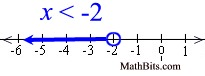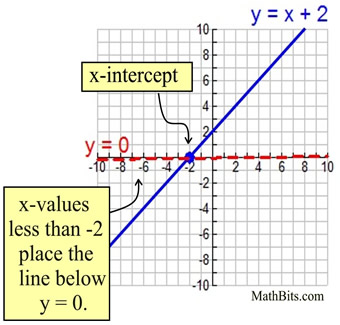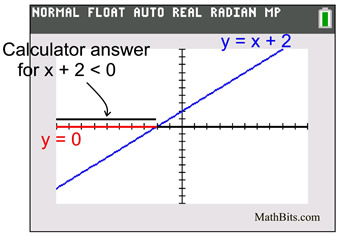Number Lines and Coordinate Axes MathBitsNotebook.com Terms of Use   Contact Person: Donna RobertsWe have seen how linear inequalities are graphed on a number line.
Let's take a look at how those number line graphs correspond to coordinate axes graphs.Solve x + 2 < 0
We know that this inequality simplifies to x < -2, and that its number line graph looks like:Now, let's look at its connection to the two-variable graph y = x + 2.

 The inequality x + 2 < 0 can also be thought of as asking "when is the line y = x + 2 below (less than) the line y = 0. Since y = 0 is the x-axis, this example is looking for when the line y = x + 2 is below (less than) the x-axis. The x-intercept occurs when x = -2, and the line y = x + 2 is below y = 0 to the left of the point (-2,0). The solution is the x-values less than -2 (written x < -2). A graphing calculator displays inequalities in this same manner.The graphing calculator plots a y-value of "1" for all of the x-values that will make the inequality true, and a "0" for all of the x-values that will make the inequality false. You may not see the "0" plots since they lie on top of the x-axis. The graphing calculator will not place an open circle at x = -2, but the table feature will show that the x = -2 is not a solution.

Understanding the connection between number line graphs and their associated coordinate axes graphs will come in handy when working with quadratic inequalities.